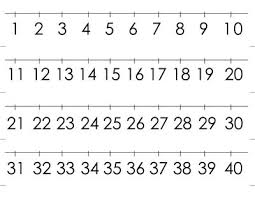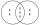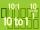# Counting number

What is the smallest counting number divisible by 2,5,7,8 and 15?

x =  840

### Step-by-step explanation:Did you find an error or inaccuracy? Feel free to write us. Thank you!Tips to related online calculators
Do you want to calculate least common multiple two or more numbers?

## Related math problems and questions:

• Lcm 2Create the smallest possible number that is divisible by numbers 5,8,9,4,3
• Find unknown numberWhat is the number between 50 and 55 that is divisible by 2,3,6,9?
• Lcm of three numbersWhat is the Lcm of 120 15 and 5
• LCM of two numberFind the smallest multiple of 63 and 147
• Reminder and quotientThere are given numbers A = 135, B = 315. Find the smallest natural number R greater than 1 so that the proportions R:A, R:B are with the remainder 1.
• The smallest numberWhat is the smallest number that can be divided by both 5 and 7
• LCMWhat is the least common multiple of 5, 50, 14?
• DecomposeDecompose into primes and find the smallest common multiple n of (16,20) and the largest common divisor D of the pair of numbers (140,100)
• MO C–I–1 2018An unknown number is divisible by just four numbers from the set {6, 15, 20, 21, 70}. Determine which ones.
• Apples and pearsMom divided 24 apples and 15 pears to children. Each child received the same number of apples and pears - same number as his siblings. How many apples (j=?) and pears (h=?) received each child?
• Lcm simpleFind least common multiple of this two numbers: 140 175.
• A number 5A number is divisible by 24, 25, and 120 if it is increased by 20. Find the number.
• FlowerbedsWhat is the smallest length of flowerbeds we have to prepare to plant seedlings at grid 20,30,25,40 cm?
• LCMCommon multiple of three numbers is 3276. One number is in this number 63 times, second 7 times, third 9 times. What are the numbers?
• A ropeA rope can be cut into equal length with no rope left over. The lengths can be 15cm,18cm or 25cm. What is the shortest possible length of the rope?
• SchoolHeadteacher think whether the distribution of pupils in race in groups of 4,5,6,9 or 10. How many pupils must have at least school at possible options?
• PlumsIn the bowl are plums. How many would be there if we can divide it equally among 8, 10 and 11 children?